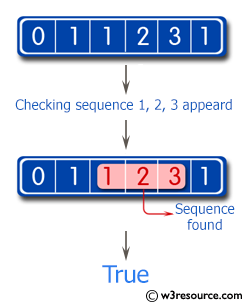﻿ Swift Basic Programming Exercise: Test if the sequence of numbers 1, 2, 3 appears in a given array of integers somewhere - w3resource# Swift Basic Programming Exercise: Test if the sequence of numbers 1, 2, 3 appears in a given array of integers somewhere

## Swift Basic Programming: Exercise-24 with Solution

Write a Swift program to test if the sequence of numbers 1, 2, 3 appears in a given array of integers somewhere.

Pictorial Presentation:Sample Solution:

Swift Code:

``````func array012(_ input: [Int]) -> Bool {
for (index, number) in input.enumerated() {
let third_Index = index + 2
let second_Index = index + 1

if second_Index < input.endIndex && number == 1 && input[second_Index] == 2 && input[third_Index] == 3 {
return true
}
}
return false
}

print(array012([0, 1, 1, 2, 3, 1]))
print(array012([0, 1, 1, 2, 4, 1]))
print(array012([1, 1, 2, 0, 1, 2, 3]))
```
```

Sample Output:

```true
false
true
```

Swift Programming Code Editor:

Improve this sample solution and post your code through Disqus

What is the difficulty level of this exercise?

﻿